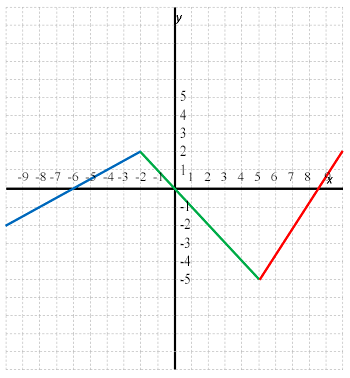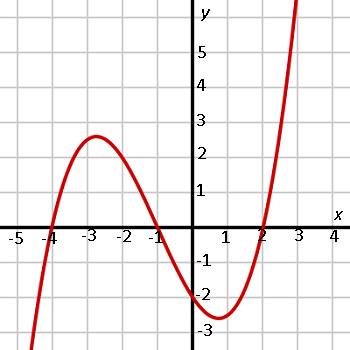# How to Interpret Mathematical Functions

Instructor: Michael Quist

Michael has taught college-level mathematics and sociology; high school math, history, science, and speech/drama; and has a doctorate in education.

Interpreting a mathematical function means obtaining meaningful information from a line graph or given symbolic representation. In this lesson, we will explore how to obtain meaningful information from graphs and function formulas.

## What is a Mathematical Function?

A mathematical function consists of three parts: input, output and the relationship between the two, which can be depicted on a graph. Interpreting a function means converting the symbols of a formula or a drawn graph into meaningful information that fits what you're looking for. When you're interpreting a function, you're answering questions based on the occasionally cryptic information available.## Interpreting a Function Graph

For example, perhaps we want to answer the following questions about the three-region graph pictured above:

1. What type of function is it?
2. What are the domain and range?
3. At what points does it cross the x and y axes?
4. At what parts it the y value increasing as x increases (positive slope)?

Looking at the graph, we can figure out that:

1. It's a piece function. There are three different sections (shown in different colors in the picture to help you visualize them), which behave in different ways. Each break in the flow of the line tells you that the formula has changed at that point. In addition, each of the three sections is a straight line, which means you're not dealing with any exponents larger than 1.
2. The domain is the set of possible x values. In this function, the domain appears to extend from x = -10 (left side of the graph) to x = 10 (right side of the graph). If a picture shows only part of the graph, as is often the case, then the domain might be much larger. The highest point on the graph shown above is y = 2, and the lowest point is y = -5, so that establishes the range. Again, there may be more to the line, which would affect the range.
3. The line crosses the x axis at approximately x = -6, x = 0, and x = 8.5. It appears to cross the y axis at y = 0.
4. The line climbs the left side of the graph (x = -10) until it reaches x = -2, where it changes direction. It also appears to increase between x = 5 and x = 10.

## Steps for Interpreting Graphs

### Determine the Type.

The function type will tell you what kind of formula or relationship you're working with and the general behavior you can expect from the function.### Determine Key Points

Maximum points, minimum points, domain, range, x intercepts, y intercepts, and end behavior (which directions do the ends of the graph go?) are all useful pieces of information toward understanding the function.

In the 3rd degree polynomial graph above, we can see that the function appears to have a local maximum at x = -.8 and a local minimum at x = 2.8. The domain and range may be infinite, since the graph probably continues beyond what is shown. The function appears to cross the x axis at x = -2, x = 1, and x = 4. It appears to cross the y axis at y = 2. The end appears to extend toward negative infinity (downward) on the left and positive infinity (upward) on the right of the graph.

### Determine the Equation, Formula, or Definition

In the 3rd degree graph shown, the places where it crosses the x axis are keys to the formula. We know that at those values for x, the function output goes to 0. This means the graph will be some version of the polynomial you get when you multiply three binomials together:

(x + 2)(x - 1)(x - 4) = x³ + x² - 6x + 8

To unlock this lesson you must be a Study.com Member.

### Register to view this lesson

Are you a student or a teacher?

### Unlock Your Education

#### See for yourself why 30 million people use Study.com

##### Become a Study.com member and start learning now.
Back
What teachers are saying about Study.com

### Earning College Credit

Did you know… We have over 160 college courses that prepare you to earn credit by exam that is accepted by over 1,500 colleges and universities. You can test out of the first two years of college and save thousands off your degree. Anyone can earn credit-by-exam regardless of age or education level.